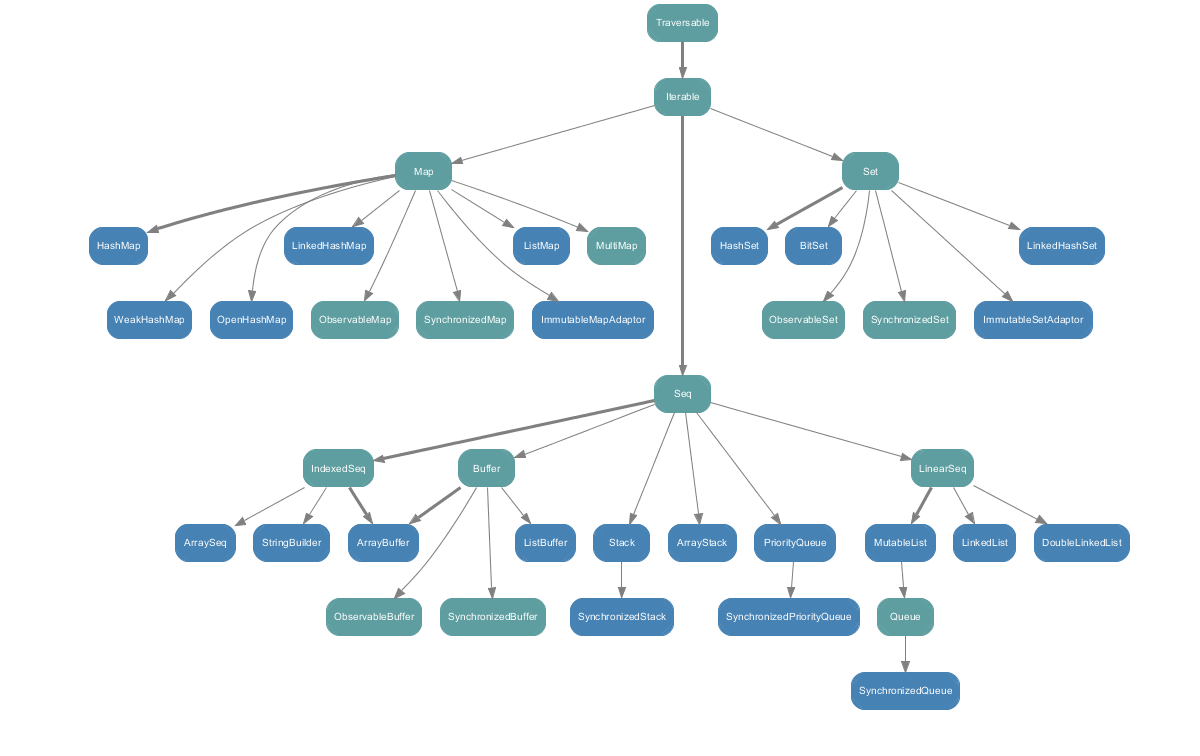# Scala primer

This Scala primer is not intended as a study material for someone new to Scala but as a refresher for someone who hasn't worked on the technology for a while.

• If the type parameter starts with a lowercase then it means the type parameter is unknown. it is semantically equal to a type parameter as underscore. ie `ClassName[t] == ClassName[_]`. use this if you want to use the classname without specifying the type parameter.

• == operator (which is a method) simply calls equals which is logical equality. there is a separate method called eq which looks for object identity.

• empty parantheses () is a synonym for Unit data type. Unlike other languages like Python empty data is not Falsy. ie `"" != false`

• Tuple is not a collection in Scala and they can no more then 22 elements. And since it is not a collection it is not iterable.

• `{ expression block }` can contain a series of expression and it returns the last expression. It can be used inlieu of a single expression. expression blocks can be nested too.

• for an if statement without the else counterpart, on failure condition the return type is Unit (descendant of AnyVal). This explains the below.
```scala> val a = if (true) { 10 } // LHS is AnyVal and not Int
a: AnyVal = 10

scala> val a = if (true) { "" } // LHS is Any and not String
a: Any = ""
```

• case match supports multiple patterns with Pipe operator.
```scala> val kind = day match {
case "MON" | "TUE" | "WED" | "THU" | "FRI" =>
"weekday"
case "SAT" | "SUN" =>
"weekend"
}
kind: String = weekday
```

• procedure is a function that has a return type of Unit. which means it a fully side-effecting function. A function definition without the equal sign means it is a procedure and would ignore the last expression and always return Unit data type.

• if a function is defined without paramaters/parentheses then it has to be called without parentheses. i.e. def test = { } has to be called `test` and `test()` is not allowed. in these cases if you want to access the function object without invoking the function then you will have to do something like `val a = greet _`. Here you are creating a partially applied function for greet. Another alternative here is to explicitly set the type of val a. ie. val a:Int=>String = greet.similarly consider this curried function `def add(a:Int)(b:Int)`. now to create a partially applied function you would have make the call as something like this `val a = add(10)(_)`.

• `@annotation.tailrec` use this annotation to ensure tail recursion in a function. If compiler cannot enforce tail recursion optimization then a compile time failure is launched.

• varargs parameter are supported. eg: `def test(a:Int*)`.

• Even type parameter of a function is optional if it can be inferred. for eg: `def identity[A](a:A) = a can be called as val a = identity(“string”).` here both the type parameter of the function and as well as the return type of val a are both inferred.

• The infix or operator notation famous for one argument object methods like `a + b = a.+(b)` can also be used for methods with two parameters like this `a + (b,c) = a.+(b,c)`

• A function with single parameter can be expressed with type `Int => Int`. But function with no parameters require an empty parentheses `() => Int`.

• function definition and literal forms
``` // one parameter example
def act(s:String,f:String=>String) = f(s)
act("Hello",(a:String) => a.reverse) full form
act("Hello",a => a.reverse) shorter type inferred form
act("Hello",_.reverse) placeholder form
// two parameter example
def act(s1:String,s2:String,f:(String,String)=>String) = f(s1,s2)
act("Hello","World",(a:String,b:String) => a+b)
act("Hello","World",(a,b) => a+b)
act("Hello","World",_+_)
```

• Partial applied functions can be created with placeholder syntax. for eg
```def add(a:Int,b:Long) = a+b
p1(20)
p2(30)
```

Here the palceholder parameters requires explicit type since there is chance of ambiguity due to overloaded method. ie we must unambigously select add(Int,Int) instead of a potential overloaded method add(Int,String)

• By-name function parameters are denoted with an additional arrow like `def func1(a: => Int) = println(a)`. func1 is not a higher order function that takes function value as argument that returns int as the function definition seems to suggest. The argument can either be a function that returns an Int or can be a direct value Int. if it is a function then the function is evaluated (ie called) everytime when the function is referenced in the func1 method body.
```def func1(a: => Int) = println(a)
val a = () => 10
val b = (a:Int) => a
func1(a()) # prints 10
func1(b(10)) # prints 10
func1(10) # prints 10
```

• Partially applied functions and Partial functions are two different things in Scala. former is a function which has currently defined only a partial list of parameters and yet to define the other parameters. The latter is a function which has restrictions on the values it can accept for the defined parameters. A Partial Function object must have two methods `apply` and `isDefinedAt` defined.

• a case block statement without a match is a partial function literal. `{ case i: Int if i != 0 => i}` is equivalent to `new PartialFunction[Int,Int] { def apply(a:Int) = a; def isDefinedAt(a:Int) = a!=0 }`

• Nil is singleton instance of List[Nothing]. And since Nothing is a subtype of every other type Nil is an empty list of any type

• apply,unapply and update are special methods in scala. if class called Test had these methods defined on them then

apply => called on Test()

unapply => called when used with pattern matching

update => called on assignment Test(10) = “Some Value”

• The Scala collections hierarchy as shown below.